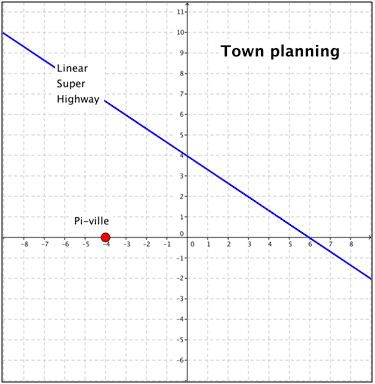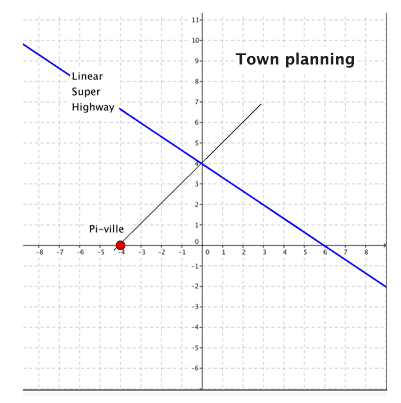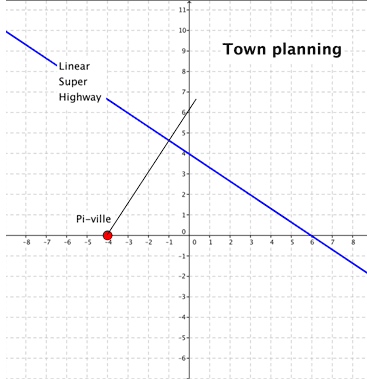# Creating an equation that models a scenario

• Kirito123

## Homework Statement

5.The small town of Pi-ville wants to construct a road that connects to the Linear Super Highway. The road must provide a route that covers the shortest distance possible from the town to the highway. Write an equation that models the best route for the road.y=mx+b

## The Attempt at a Solution

ok so I am guessing that i have to draw a line from Pi-ville to the linear super highway. Then determine the slope and y-intercept of that line to develop an equation that represent the best route for the road.
i chose to draw a line from pi-ville to the point (0,4) on the linear highway.then i calculated the slope of the line and determined the y-intercept

(-4, 0) (0, 4)
slope = (y2-y1) /(x2-x1)
slope = (4-0) / 0-(-4)
slope = 4/4 = 1

y-intercept: +4 (since the line crosses the vertical axis at point 4)

an equation to represent the best route for the road is:
y=mx + b
y = 4/4x + 4

is this right?

My guess reading is that here 'best' means 'shortest'. What is the characteristic of the shortest path to the blue line ?

do you mean that there is a path from pi-ville to the linear highway that is shorter than the path i chose?

do you mean that there is a path from pi-ville to the linear highway that is shorter than the path i chose?

He does mean precisely that!is this a shorter route?

(-4, 0) (-1,4.8)
slope = (y2-y1) /(x2-x1)
slope = (4.8-0) / (-1 -(-4)) = 4.8/3

y-intercept: +6 (since the line crosses the vertical axis at point 6)

an equation to represent the best route for the road is:
y=mx + b
y = 4.8/3x + 6

right?

(-4, 0) (-1,4.8)
slope = (y2-y1) /(x2-x1)
slope = (4.8-0) / (-1 -(-4)) = 4.8/3

y-intercept: +6 (since the line crosses the vertical axis at point 6)

an equation to represent the best route for the road is:
y=mx + b
y = 4.8/3x + 6

right?

You need to write ##(4.8/3)x##

What happens when ##y = 0##? Where is the x-intercept from your equation?

i got -3.75 after substituting 0 in for y in my equation?

i got -3.75 after substituting 0 in for y in my equation?

Yes, but it should be ##-4##.

so what does -4 represent?

so what does -4 represent?

The x-intercept you can see on your diagram!

ooh i get it, so does this mean my answer is correct, or is it a few decimal places off, since i got -3.75 instead of -4

ooh i get it, so does this mean my answer is correct, or is it a few decimal places off, since i got -3.75 instead of -4

I think you can safely say that your equation is not correct!

ok, so instead of using the point (-1, 4.8), i used (0, 6)
and i got the equation y=1.5x + 6
i get -4 as the x-intercept when substituting 0 in for y
im assuming this is correct?

ok, so instead of using the point (-1, 4.8), i used (0, 6)
and i got the equation y=1.5x + 6
i get -4 as the x-intercept when substituting 0 in for y
im assuming this is correct?

That looks better.

thanks for the help :)